# ISEE Upper Level Quantitative : Variables

## Example Questions

### Example Question #41 : Operations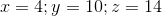Which of the following is the greater quantity?

(A)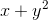(B)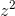(A) is greater

(B) is greater

It is impossible to determine which is greater from the information given

(A) and (B) are equal

(B) is greater

Explanation:

(A) Substitute and evaluate, remembering that by order of operations, squaring precedes addition: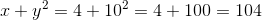(B) Substitute and evaluate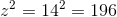(B) is greater

### Example Question #42 : Operationsis negative. Which of these quantities is the greater?

(A)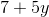(B)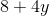(A) is greater

It is impossible to determine which is greater from the information given

(A) and (B) are equal

(B) is greater

(B) is greater

Explanation:

Since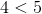andis negative, then by the multiplication property of inequality,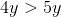Also,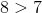, so by the addition property of inequality,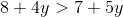making (B) greater.

### Example Question #41 : Variablesis positive. Which of these quantities is greater?

(A)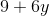(B)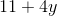It is impossible to determine which is greater from the information given

(B) is greater

(A) is greater

(A) and (B) are equal

It is impossible to determine which is greater from the information given

Explanation:

The values of both terms depend on the value of.

Case 1: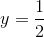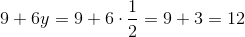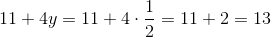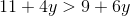, making (B) greater.

Case 2: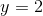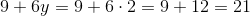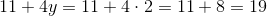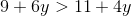, making (A) greater.

Therefore, the information given is insufficient.

### Example Question #44 : Operations

When evaluating the expression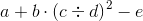,

assuming you know the values of all five variables, what is the third operation that must be performed?

Multiplication

Squaring

Division

Subtraction

Multiplication

Explanation:

The operation that must be performed first is the operation in parentheses, which is the division.

After this, the order of operations is:

Exponents

Multiplication and division (left to right)

Addition and subtraction (left to right)

The second thing that must be done is the exponentiation - the squaring. Of the three remaining operations, the multiplication takes precedence. The correct response is multiplication.

### Example Question #42 : Variables

The Fibonacci sequence is defined as follows: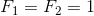For integers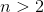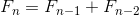.

Which is the greater quantity?

(a) The sum of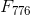and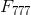(b)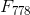(a) and (b) are equal

It is impossible to determine which is greater from the information given

(a) is greater

(b) is greater

(a) and (b) are equal

Explanation:

Using the definition of the sequence for all terms beginning with the third, substitute 778 for: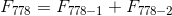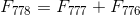The two quantities are equal.

### Example Question #11 : How To Add Variablesis a negative integer. Which is the greater quantity?

(A)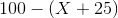(B)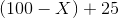(a) and (b) are equal

It cannot be determined which of (a) and (b) is greater

(b) is the greater quantity

(a) is the greater quantity

(b) is the greater quantity

Explanation: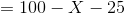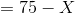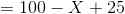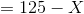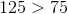, so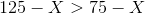andis the greater quantity, regardless of.

### Example Question #12 : How To Add Variablesis a positive number.

Which is the greater quantity?

(a)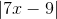(b)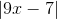(a) is the greater quantity

(a) and (b) are equal

(b) is the greater quantity

It cannot be determined which of (a) and (b) is greater

It cannot be determined which of (a) and (b) is greater

Explanation:

By examining two scenarios, we see that we cannot determine which is the greater quantity.

Case 1: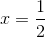Then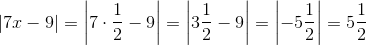and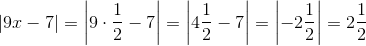This makes (a) the greater quantity.

Case 2:Then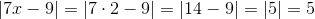and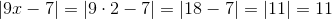This makes (b) the greater quantity.

### Example Question #48 : Operationsis a negative number.

Which is the greater quantity?

(a)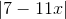(b)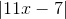It cannot be determined which of (a) and (b) is greater

(b) is the greater quantity

(a) and (b) are equal

(a) is the greater quantity

(a) and (b) are equal

Explanation: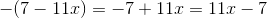The expressions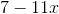and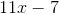are each other's opposite, so they have the same absolute value. Therefore, regardless of the value of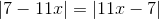.

### Example Question #13 : How To Add Variablesis a negative integer. Which is the greater quantity?

(A)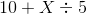(B)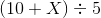It cannot be determined which of (a) and (b) is greater

(a) and (b) are equal

(b) is the greater quantity

(a) is the greater quantity

(a) is the greater quantity

Explanation: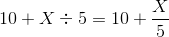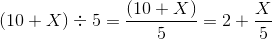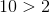,

so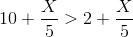and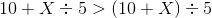regardless of the value of.

### Example Question #50 : Operationsis a negative integer. Which is the greater quantity?

(a)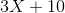(b)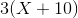It cannot be determined which of (a) and (b) is greater

(a) and (b) are equal

(b) is the greater quantity

(a) is the greater quantity

(b) is the greater quantity

Explanation: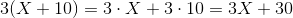Since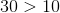,

it follows that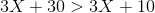and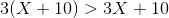regardless of the value of.

### All ISEE Upper Level Quantitative Resources